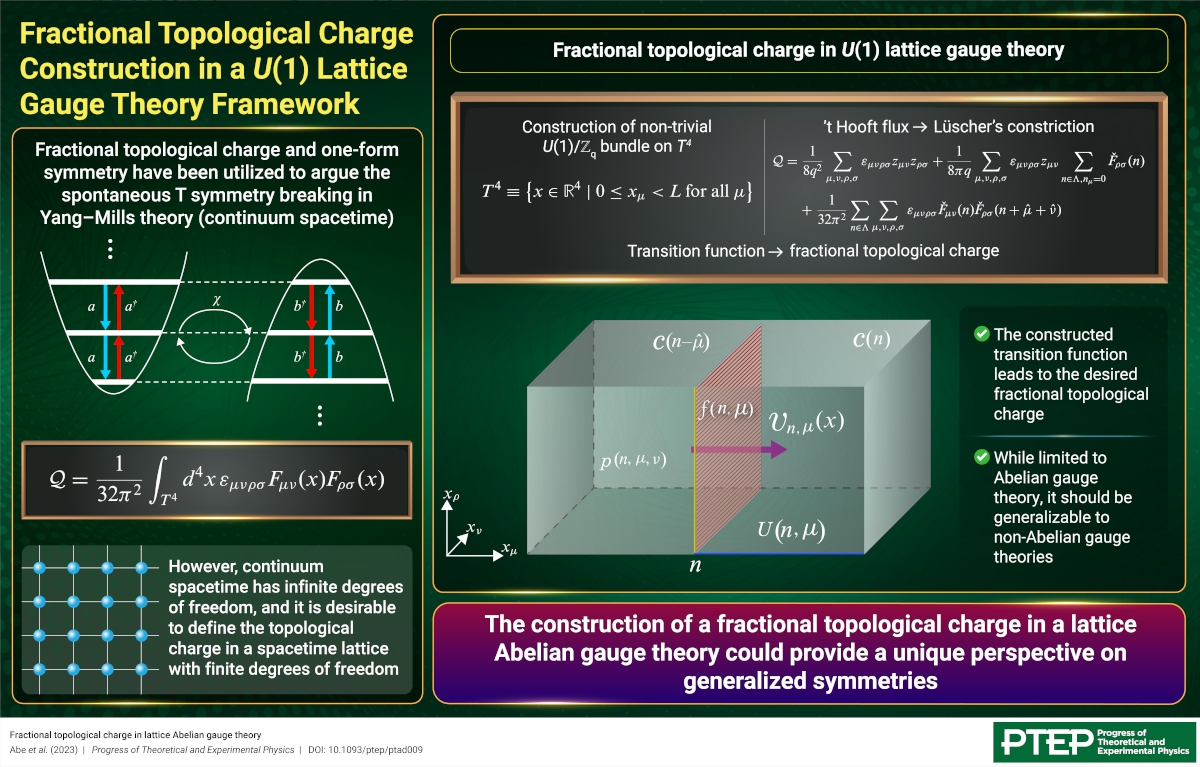# Fractional Topological Charge Construction in a U(1) Lattice Gauge Theory Framework

2023-3-27

JPS Hot Topics 3, 010

https://doi.org/10.7566/JPSHT.3.010

© The Physical Society of Japan

Fractional topological charge in lattice Abelian gauge theory
(PTEP Editors' Choice)

Motokazu Abe, Okuto Morikawa, and Hiroshi Suzuki
Prog. Theor. Exp. Phys. 2023, 023B03 (2023).

We have constructed a fractional topological charge in the lattice Abelian gauge theory formalism, which avoids the issue of infinite degrees of freedom in continuum spacetime quantum field theory.The invariance of certain features of a dynamical system under continuous or discrete transformations is a fundamental concept in physics called symmetry. Symmetry is extremely useful for studying systems in fields ranging from high energy particle physics to condensed matter physics. Given its importance, the concept has been generalized to higher forms (one-form, two-form, etc.) and non-invertible symmetries.

One particular example of the application of such generalized symmetries comes from the spontaneous breaking of time-reversal (T) symmetry in Yang-Mills theory, which uses the one-form symmetry and the anomaly associated with it. Notably, this anomaly arises from a fractional topological charge emerging from a two-form gauge field.

Such a fractional topological charge has been formulated in continuum quantum field theory. However, the formulation remains rather formal since quantum field theory, considered in continuum spacetime, contains an infinite number of degrees of freedom and is not mathematically well-defined.

A simpler and more desirable alternative is to formulate the problem in the context of lattice gauge theory, which deals with a spacetime lattice. This offers the advantages of both a finite number of degrees of freedom and a mathematically well-defined theory. However, such a construction has not been attempted so far.

Accordingly, we present, in this study, a fractional topological charge construction in U(1) lattice gauge theory. We begin by constructing the transition function of a non-trivial U(1)/Zq principle bundle on T4 from the U(1) lattice gauge field using a generalized Lüscher’s constriction. This transition function satisfies the cocycle condition of the U(1)/Zq gauge theory. The cocycle condition contains a Zq factor that corresponds to the two-form gauge field encountered in the one-form symmetry.

In the next step, we combine this transition function with the ’t Hooft flux to obtain a topological charge that takes on fractional values and is odd under lattice T transformation.

While our formulation is limited to an Abelian lattice gauge theory, it should be possible to extend it to non-Abelian gauge theories. Such a construction will provide a unique perspective on generalized symmetries with a simpler lattice spacetime implementation.

Fractional topological charge in lattice Abelian gauge theory
(PTEP Editors' Choice)

Motokazu Abe, Okuto Morikawa, and Hiroshi Suzuki
Prog. Theor. Exp. Phys. 2023, 023B03 (2023).